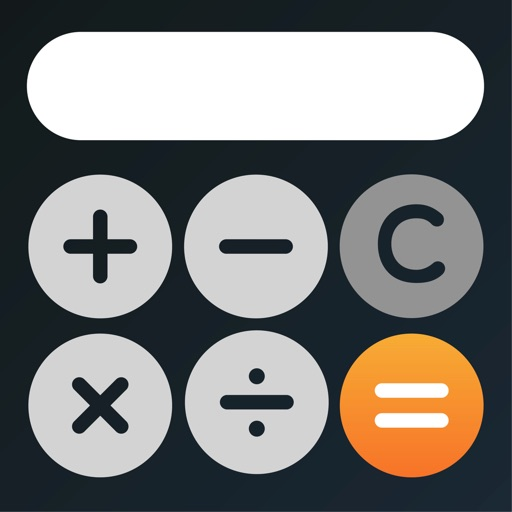# myCalc: Calculator +

myCalc is a simple to use basic & scientific calculator with great advanced options for the most demanding users!
Category Price Seller Device
Productivity Free Appgeneration, Software Technologies, Lda iPhone, iPad, iPod

Use it in portrait mode or landscape mode if you need some extra functions. You can also use myCalc for up-to-date currency conversions and unit conversions.

You can choose between our special themes for a more customized experience.

FEATURES

- Basic and scientific calculators
- History: copy result / conversion / equation and share
- Memory banks
- Calculate with degrees or radians
- Currency converter between 30+ currencies
- Unit converter between 60+ measures
- 10+ unit types: length, area, volume, temperature, ...
- Set the number of decimal places
- Choose the decimal notation
- Choose the digit grouping symbol
- 10+ personalized themes, including several dark themes
- Portrait and landscape mode
- Compatible with iPad split view

MATHEMATICAL OPERATIONS

Addition, subtraction, multiplication, division, percentage, square root, cubic root, nth root, square, cubic exponent, nth exponent, engineering exponent, factorial power of two, power of ten, power of E natural logarithm, common logarithm, binary logarithm, sine, cosine, tangent, arcsine, arccosine, arctangent, hyperbolic sine, hyperbolic cosine, hyperbolic tangent, hyperbolic arcsine, hyperbolic arc cosine, hyperbolic arctangent, multiplicative inverse, and random number input.

UNIT TYPES

Area, volume, energy, fuel efficiency, length, power, pressure, speed, temperature, and weight.

myCalc gives you an accurate and fast answer to your math problems with a beautiful look!